# 12.4 Rotation of axes  (Page 5/8)

 Page 5 / 8

Identify the conic for each of the following without rotating axes.

1. ${x}^{2}-9xy+3{y}^{2}-12=0$
2. $10{x}^{2}-9xy+4{y}^{2}-4=0$
1. hyperbola
2. ellipse

Access this online resource for additional instruction and practice with conic sections and rotation of axes.

## Key equations

 General Form equation of a conic section $A{x}^{2}+Bxy+C{y}^{2}+Dx+Ey+F=0$ Rotation of a conic section Angle of rotation

## Key concepts

• Four basic shapes can result from the intersection of a plane with a pair of right circular cones connected tail to tail. They include an ellipse, a circle, a hyperbola, and a parabola.
• A nondegenerate conic section has the general form $\text{\hspace{0.17em}}A{x}^{2}+Bxy+C{y}^{2}+Dx+Ey+F=0\text{\hspace{0.17em}}$ where $\text{\hspace{0.17em}}A,B\text{\hspace{0.17em}}$ and $\text{\hspace{0.17em}}C\text{\hspace{0.17em}}$ are not all zero. The values of $\text{\hspace{0.17em}}A,B,$ and $\text{\hspace{0.17em}}C\text{\hspace{0.17em}}$ determine the type of conic. See [link] .
• Equations of conic sections with an $\text{\hspace{0.17em}}xy\text{\hspace{0.17em}}$ term have been rotated about the origin. See [link] .
• The general form can be transformed into an equation in the $\text{\hspace{0.17em}}{x}^{\prime }\text{\hspace{0.17em}}$ and $\text{\hspace{0.17em}}{y}^{\prime }\text{\hspace{0.17em}}$ coordinate system without the $\text{\hspace{0.17em}}{x}^{\prime }{y}^{\prime }\text{\hspace{0.17em}}$ term. See [link] and [link] .
• An expression is described as invariant if it remains unchanged after rotating. Because the discriminant is invariant, observing it enables us to identify the conic section. See [link] .

## Verbal

What effect does the $\text{\hspace{0.17em}}xy\text{\hspace{0.17em}}$ term have on the graph of a conic section?

The $\text{\hspace{0.17em}}xy\text{\hspace{0.17em}}$ term causes a rotation of the graph to occur.

If the equation of a conic section is written in the form $\text{\hspace{0.17em}}A{x}^{2}+B{y}^{2}+Cx+Dy+E=0\text{\hspace{0.17em}}$ and $\text{\hspace{0.17em}}AB=0,$ what can we conclude?

If the equation of a conic section is written in the form $\text{\hspace{0.17em}}A{x}^{2}+Bxy+C{y}^{2}+Dx+Ey+F=0,$ and $\text{\hspace{0.17em}}{B}^{2}-4AC>0,$ what can we conclude?

The conic section is a hyperbola.

Given the equation $\text{\hspace{0.17em}}a{x}^{2}+4x+3{y}^{2}-12=0,$ what can we conclude if $\text{\hspace{0.17em}}a>0?$

For the equation $\text{\hspace{0.17em}}A{x}^{2}+Bxy+C{y}^{2}+Dx+Ey+F=0,$ the value of $\text{\hspace{0.17em}}\theta \text{\hspace{0.17em}}$ that satisfies $\text{\hspace{0.17em}}\mathrm{cot}\left(2\theta \right)=\frac{A-C}{B}\text{\hspace{0.17em}}$ gives us what information?

It gives the angle of rotation of the axes in order to eliminate the $\text{\hspace{0.17em}}xy\text{\hspace{0.17em}}$ term.

## Algebraic

For the following exercises, determine which conic section is represented based on the given equation.

$9{x}^{2}+4{y}^{2}+72x+36y-500=0$

${x}^{2}-10x+4y-10=0$

$AB=0,$ parabola

$2{x}^{2}-2{y}^{2}+4x-6y-2=0$

$4{x}^{2}-{y}^{2}+8x-1=0$

$AB=-4<0,$ hyperbola

$4{y}^{2}-5x+9y+1=0$

$2{x}^{2}+3{y}^{2}-8x-12y+2=0$

$AB=6>0,$ ellipse

$4{x}^{2}+9xy+4{y}^{2}-36y-125=0$

$3{x}^{2}+6xy+3{y}^{2}-36y-125=0$

${B}^{2}-4AC=0,$ parabola

$-3{x}^{2}+3\sqrt{3}xy-4{y}^{2}+9=0$

$2{x}^{2}+4\sqrt{3}xy+6{y}^{2}-6x-3=0$

${B}^{2}-4AC=0,$ parabola

$-{x}^{2}+4\sqrt{2}xy+2{y}^{2}-2y+1=0$

$8{x}^{2}+4\sqrt{2}xy+4{y}^{2}-10x+1=0$

${B}^{2}-4AC=-96<0,$ ellipse

For the following exercises, find a new representation of the given equation after rotating through the given angle.

$3{x}^{2}+xy+3{y}^{2}-5=0,\theta =45°$

$4{x}^{2}-xy+4{y}^{2}-2=0,\theta =45°$

$7{{x}^{\prime }}^{2}+9{{y}^{\prime }}^{2}-4=0$

$2{x}^{2}+8xy-1=0,\theta =30°$

$-2{x}^{2}+8xy+1=0,\theta =45°$

$3{{x}^{\prime }}^{2}+2{x}^{\prime }{y}^{\prime }-5{{y}^{\prime }}^{2}+1=0$

$4{x}^{2}+\sqrt{2}xy+4{y}^{2}+y+2=0,\theta =45°$

For the following exercises, determine the angle $\text{\hspace{0.17em}}\theta \text{\hspace{0.17em}}$ that will eliminate the $\text{\hspace{0.17em}}xy\text{\hspace{0.17em}}$ term and write the corresponding equation without the $\text{\hspace{0.17em}}xy\text{\hspace{0.17em}}$ term.

${x}^{2}+3\sqrt{3}xy+4{y}^{2}+y-2=0$

$\theta ={60}^{\circ },11{{x}^{\prime }}^{2}-{{y}^{\prime }}^{2}+\sqrt{3}{x}^{\prime }+{y}^{\prime }-4=0$

$4{x}^{2}+2\sqrt{3}xy+6{y}^{2}+y-2=0$

$9{x}^{2}-3\sqrt{3}xy+6{y}^{2}+4y-3=0$

$\theta ={150}^{\circ },21{{x}^{\prime }}^{2}+9{{y}^{\prime }}^{2}+4{x}^{\prime }-4\sqrt{3}{y}^{\prime }-6=0$

$-3{x}^{2}-\sqrt{3}xy-2{y}^{2}-x=0$

$16{x}^{2}+24xy+9{y}^{2}+6x-6y+2=0$

$\theta \approx {36.9}^{\circ },125{{x}^{\prime }}^{2}+6{x}^{\prime }-42{y}^{\prime }+10=0$

${x}^{2}+4xy+4{y}^{2}+3x-2=0$

${x}^{2}+4xy+{y}^{2}-2x+1=0$

$\theta ={45}^{\circ },3{{x}^{\prime }}^{2}-{{y}^{\prime }}^{2}-\sqrt{2}{x}^{\prime }+\sqrt{2}{y}^{\prime }+1=0$

$4{x}^{2}-2\sqrt{3}xy+6{y}^{2}-1=0$

## Graphical

For the following exercises, rotate through the given angle based on the given equation. Give the new equation and graph the original and rotated equation.

$y=-{x}^{2},\theta =-{45}^{\circ }$

$\frac{\sqrt{2}}{2}\left({x}^{\prime }+{y}^{\prime }\right)=\frac{1}{2}{\left({x}^{\prime }-{y}^{\prime }\right)}^{2}$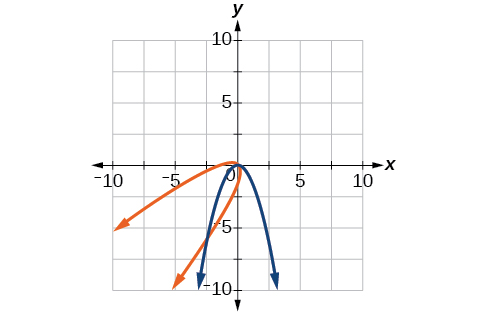$x={y}^{2},\theta ={45}^{\circ }$

$\frac{{x}^{2}}{4}+\frac{{y}^{2}}{1}=1,\theta ={45}^{\circ }$

$\frac{{\left({x}^{\prime }-{y}^{\prime }\right)}^{2}}{8}+\frac{{\left({x}^{\prime }+{y}^{\prime }\right)}^{2}}{2}=1$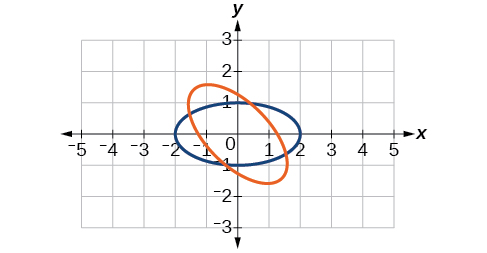$\frac{{y}^{2}}{16}+\frac{{x}^{2}}{9}=1,\theta ={45}^{\circ }$

${y}^{2}-{x}^{2}=1,\theta ={45}^{\circ }$

$\frac{{\left({x}^{\prime }+{y}^{\prime }\right)}^{2}}{2}-\frac{{\left({x}^{\prime }-{y}^{\prime }\right)}^{2}}{2}=1$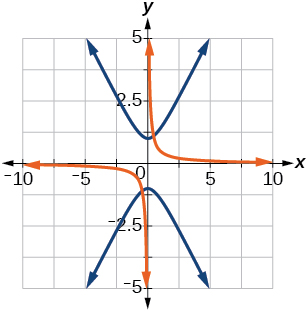$y=\frac{{x}^{2}}{2},\theta ={30}^{\circ }$

$x={\left(y-1\right)}^{2},\theta ={30}^{\circ }$

$\frac{\sqrt{3}}{2}{x}^{\prime }-\frac{1}{2}{y}^{\prime }={\left(\frac{1}{2}{x}^{\prime }+\frac{\sqrt{3}}{2}{y}^{\prime }-1\right)}^{2}$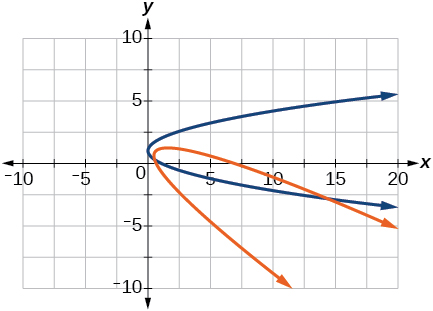$\frac{{x}^{2}}{9}+\frac{{y}^{2}}{4}=1,\theta ={30}^{\circ }$

For the following exercises, graph the equation relative to the $\text{\hspace{0.17em}}{x}^{\prime }{y}^{\prime }\text{\hspace{0.17em}}$ system in which the equation has no $\text{\hspace{0.17em}}{x}^{\prime }{y}^{\prime }\text{\hspace{0.17em}}$ term.

$xy=9$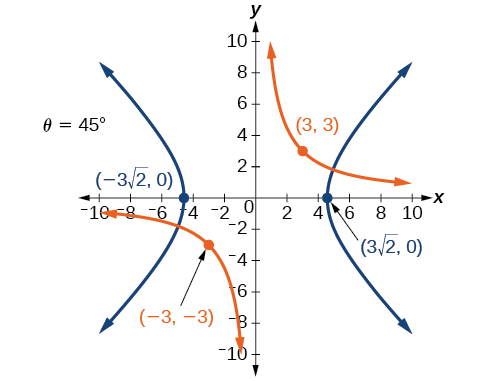${x}^{2}+10xy+{y}^{2}-6=0$

${x}^{2}-10xy+{y}^{2}-24=0$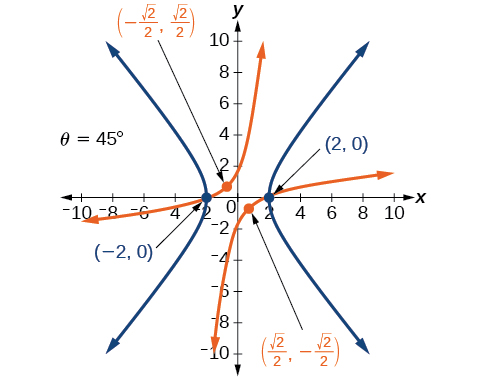$4{x}^{2}-3\sqrt{3}xy+{y}^{2}-22=0$

$6{x}^{2}+2\sqrt{3}xy+4{y}^{2}-21=0$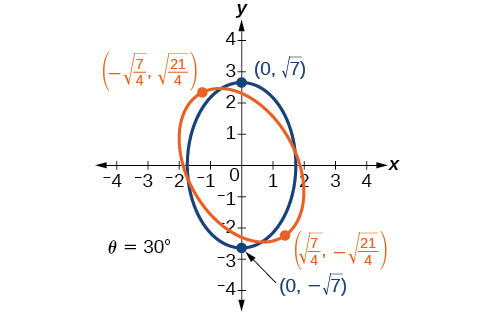$11{x}^{2}+10\sqrt{3}xy+{y}^{2}-64=0$

$21{x}^{2}+2\sqrt{3}xy+19{y}^{2}-18=0$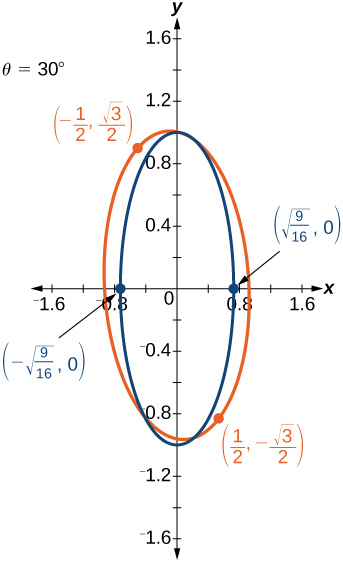$16{x}^{2}+24xy+9{y}^{2}-130x+90y=0$

$16{x}^{2}+24xy+9{y}^{2}-60x+80y=0$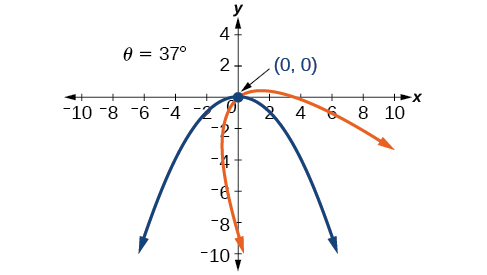$13{x}^{2}-6\sqrt{3}xy+7{y}^{2}-16=0$

$4{x}^{2}-4xy+{y}^{2}-8\sqrt{5}x-16\sqrt{5}y=0$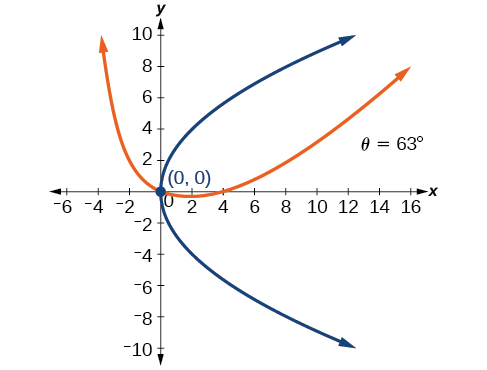For the following exercises, determine the angle of rotation in order to eliminate the $\text{\hspace{0.17em}}xy\text{\hspace{0.17em}}$ term. Then graph the new set of axes.

$6{x}^{2}-5\sqrt{3}xy+{y}^{2}+10x-12y=0$

$6{x}^{2}-5xy+6{y}^{2}+20x-y=0$

$\theta ={45}^{\circ }$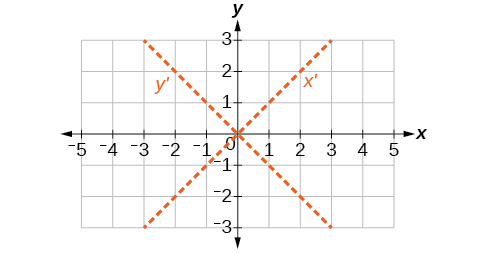$6{x}^{2}-8\sqrt{3}xy+14{y}^{2}+10x-3y=0$

$4{x}^{2}+6\sqrt{3}xy+10{y}^{2}+20x-40y=0$

$\theta ={60}^{\circ }$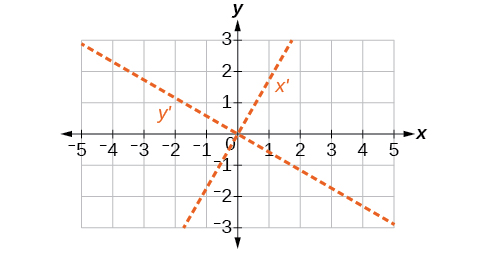$8{x}^{2}+3xy+4{y}^{2}+2x-4=0$

$16{x}^{2}+24xy+9{y}^{2}+20x-44y=0$

$\theta \approx {36.9}^{\circ }$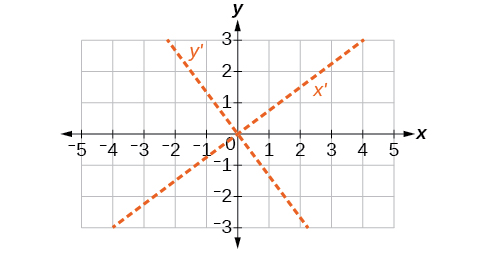For the following exercises, determine the value of $\text{\hspace{0.17em}}k\text{\hspace{0.17em}}$ based on the given equation.

Given $\text{\hspace{0.17em}}4{x}^{2}+kxy+16{y}^{2}+8x+24y-48=0,$ find $\text{\hspace{0.17em}}k\text{\hspace{0.17em}}$ for the graph to be a parabola.

Given $\text{\hspace{0.17em}}2{x}^{2}+kxy+12{y}^{2}+10x-16y+28=0,$ find $\text{\hspace{0.17em}}k\text{\hspace{0.17em}}$ for the graph to be an ellipse.

$-4\sqrt{6}

Given $\text{\hspace{0.17em}}3{x}^{2}+kxy+4{y}^{2}-6x+20y+128=0,$ find $\text{\hspace{0.17em}}k\text{\hspace{0.17em}}$ for the graph to be a hyperbola.

Given $\text{\hspace{0.17em}}k{x}^{2}+8xy+8{y}^{2}-12x+16y+18=0,$ find $\text{\hspace{0.17em}}k\text{\hspace{0.17em}}$ for the graph to be a parabola.

$k=2$

Given $\text{\hspace{0.17em}}6{x}^{2}+12xy+k{y}^{2}+16x+10y+4=0,$ find $\text{\hspace{0.17em}}k\text{\hspace{0.17em}}$ for the graph to be an ellipse.

the third and the seventh terms of a G.P are 81 and 16, find the first and fifth terms.
if a=3, b =4 and c=5 find the six trigonometric value sin
pls how do I factorize x⁴+x³-7x²-x+6=0
in a function the input value is called
how do I test for values on the number line
if a=4 b=4 then a+b=
a+b+2ab
Kin
commulative principle
a+b= 4+4=8
Mimi
If a=4 and b=4 then we add the value of a and b i.e a+b=4+4=8.
Tariq
what are examples of natural number
an equation for the line that goes through the point (-1,12) and has a slope of 2,3
3y=-9x+25
Ishaq
show that the set of natural numberdoes not from agroup with addition or multiplication butit forms aseni group with respect toaaddition as well as multiplication
x^20+x^15+x^10+x^5/x^2+1
evaluate each algebraic expression. 2x+×_2 if ×=5
if the ratio of the root of ax+bx+c =0, show that (m+1)^2 ac =b^2m
By the definition, is such that 0!=1.why?
(1+cosA+IsinA)(1+cosB+isinB)/(cos@+isin@)(cos$+isin$)
hatdog
Mark
jaks
Ryan
how we can draw three triangles of distinctly different shapes. All the angles will be cutt off each triangle and placed side by side with vertices touching

#### Get Jobilize Job Search Mobile App in your pocket Now!ByByBy RhodesBy OpenStaxBy Subramanian DivyaBy Briana KnowltonBy Angela JanuaryByBy OpenStaxBy Brooke DelaneyBy Courntey HubBy